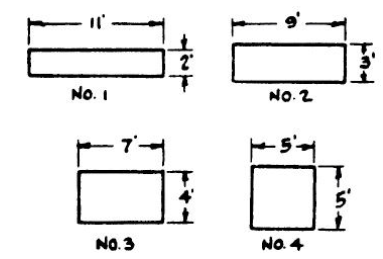# PiCAT Mechanical Comprehension Practice Test 2023

PiCAT Mechanical Comprehension Practice Test 2023: Try our free Prescreen Internet Computerized Adaptive Test (PiCAT) US Armed Forces Mechanical Comprehension review questions and answers. You can also download PiCAT Mechanical Comprehension Practice Test in PDF.

This Mechanical Comprehension test has 16 questions about mechanical principles. Most of the questions use drawings to illustrate specific principles. Choose the correct answer.

### PiCAT Mechanical Comprehension Practice Test 2023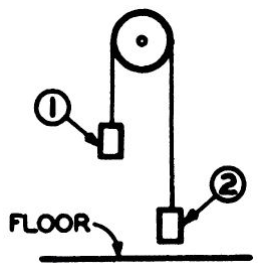Q1. The figure above represents a pulley, with practically no friction, from which two 10-pound weights are suspended as indicated. If a downward force is applied to weight 1, it is most likely that weight 1 will

• A. come to rest at the present level of weight 2.
• B. move downward until it is level with weight 2.
• C. move downward until it reaches the floor.
• D. pass weight 2 in its downward motion and then return to its present position.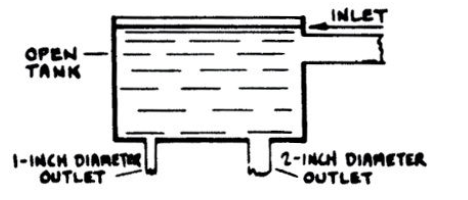Q2. Eight gallons of water per minute are flowing at a given time from the 1-inch outlet in the tank shown above. What is the amount of water flowing at that time from the 2-inch outlet?

• 2-A 64 gallons per minute
• B 32 gallons per minute
• C 16 gallons per minute
• D 2 gallons per minute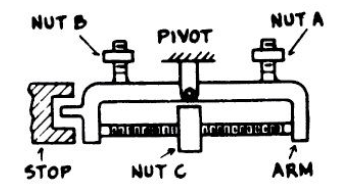Q3. The arm in the figure above is exactly balanced as shown. If nut A is removed entirely, then, in order to rebalance the arm, it will be necessary to turn nut

• A. C toward the right.
• B. C toward the left.
• C. B up.
• D. B down.

Q4. The purpose of an air valve in a heating system is to

• A. prevent pressure from building up in a room due to the heated air.
• B. relieve the air from steam radiators.
• C. allow excessive steam pressure in the boiler to escape to the atmosphere.
• D. control the temperature in the room.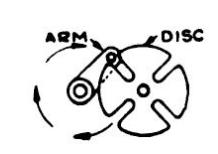Q5. The figure above shows a slotted disc turned by a pin on a rotating arm. One revolution of the arm turns the disc

• A. \frac14
• B. \frac12
• C. \frac34
• D one complete turn.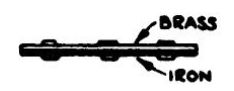Q6. The figure above shows a brass and an iron strip continuously riveted together. High temperatures would probably

• A. have no effect at all.
• B. bend the strips.
• C. separate the strips.
• D. shorten the strips.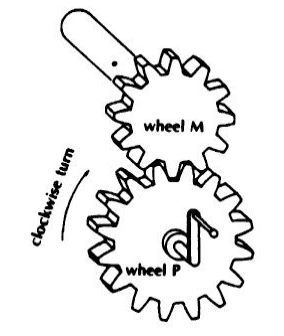Q7. Study the gear wheels in the figure above, then determine which of the following statements is true.

• A. If you turn wheel M clockwise by means of the handle, wheel P will also turn clockwise.
• B. It will take the same time for a tooth of wheel P to make a full turn as it will for a tooth of wheel M.
• C. It will take less time for a tooth of wheel P to make a full turn than it will take a tooth of wheel M.
• D. It will take more time for a tooth of wheel P to make a full turn than it will for a tooth of wheel M.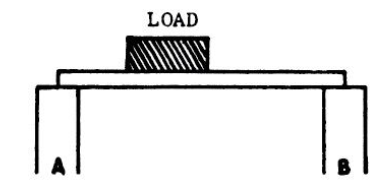Q8. In the figure above, which upright supports the greater part of the load?

• A. Upright A
• B. Upright B
• C. They support it equally.
• D. It cannot be determined.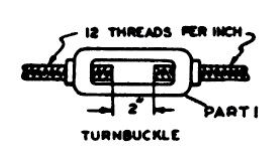Q9. For the turnbuckle shown above, the number of complete turns of Part 1 required to make the ends of the threaded rods meet is

• A. 6
• B. 18
• C. 12
• D. 24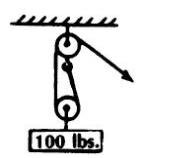Q10. When the 100-pound weight is being slowly hoisted up by the pulley, as shown in the figure above, the downward pull on the ceiling to which the pulley is attached is

• A. 50 pounds.
• B. 100 pounds.
• C. 150 pounds.
• D. 200 pounds.Q11. When the driver wheel in the figure above is moved from location X to location Y, the driven wheel will

• A. reverse its direction of rotation.
• B. turn slower.
• C. not change its speed of rotation.
• D. turn faster.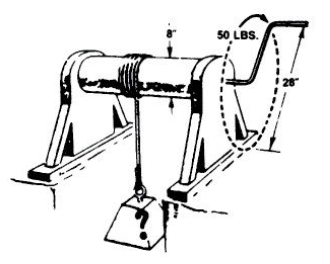Q12. In the diagram above, the axle 8 inches in diameter has attached a handle 28 inches in diameter. If a force of 50 pounds is applied to the handle, the axle will lift a weight of

• A. 224 pounds.
• B. 175 pounds.
• C. 200 pounds.
• D. 88 pounds.

Q13. The main purpose of expansion joints in steam lines is to

• A. provide for changes in length of heated pipe.
• C. provide locations for valves.
• D. reduce breakage of pipe due to minor movement of the building frame.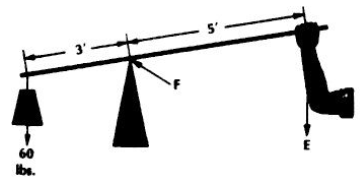Q14. What effort must be exerted to lift a 60-pound weight in the figure of a first-class lever shown above (disregard the weight of the lever in your computation)?

• A. 30 pounds
• B. 36 pounds
• C. 45 pounds
• D. 60 pounds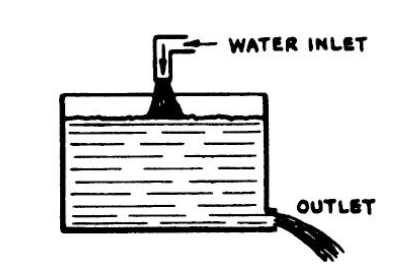Q15. If water is flowing into the tank shown above at the rate of 120 gallons per hour and flowing out of the tank at a constant rate of 1 gallon per minute, the water level in the tank will

• A. rise 1 gallon per minute.
• B. rise 2 gallons per minute.
• C. fall 2 gallons per minute.
• D. fall 1 gallon per minute.